### Home > CALC > Chapter 7 > Lesson 7.2.3 > Problem7-91

7-91.
1. Feng throws a baseball directly up in the air with an initial velocity of 16 feet per second from a height of 4 feet. Homework Help ✎

1. When will the ball hit the ground?

2. What is the ball's maximum height?

3. What is the ball's velocity when it hit the ground?

4. What is the total distance the ball traveled from t = 0 sec to t = 0.75 sec.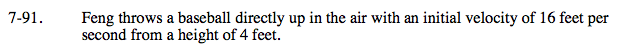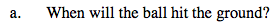Acceleration due to gravity = −32 ft/sec²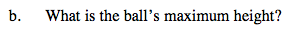Optimize. Or use Algebra to find the vertex of the parabolic position function.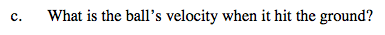First figure out WHEN it hits the ground.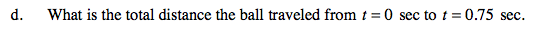Do not confuse total distance with displacement.